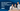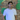Pass Any Exam Online Now & Pay After Passing Exam. Contact Now
GED Mathematical Reasoning

# GED Mathematical Reasoning: What You Need to Know

Jul 24, 2022 • 4 mins readThe GED math section is a nightmare for many students as it is considered one of the most challenging sections of the entire test. However, the good news is that you do not need a mathematical brain and hard work to pass the GED math test.

The appropriate study material and strategies are good enough to pass the GED math test. You do not have to worry about prolonged and tedious math calculations because the scientific calculator and reference formula sheets will be provided in the exam.

You just need the basic concepts, knowledge, and understanding of the topics covered in the GED test.

## What test topics are covered in the GED exam?

The GED Mathematical Reasoning section involves four significant topics:

• Basic math
• Geometry
• Basic algebra
• Graphs and functions

The GED candidates are expected to know math concepts, unit measurement, different math equations, and the application of math topics to solve real-life problems. GED candidates do not have to remember the math formulas to solve the problems as formula sheets are provided along with a scientific calculator.

## What is the format of the math test?

The GED math test is conducted in two parts in 115 min. A short break is provided between two sections, and the calculator is provided only for the second section. Candidates have access to a scientific calculator and its reference sheet as well as mathematical formula sheets. Both sections cover various types of questions: multiple choice, drag, and drop, fill in the blanks and select an area and drop and down type questions.

## How to prepare for the GED math test?

The math section is all about concepts and questions practice. You need to spend maximum time on your preparation for math questions practice. For a positive response, you are free to join classes that have huge potential to improve your scores. The experienced educator at the center or online session guides how you can efficiently prepare for the GED math test and score the highest marks with minimum effort.

Attending regular classes will be an excellent opportunity to learn math concepts in the best way.

If candidates want to practice more, they can join the GED Flash, which provides thousands of quality math questions for practice which students can access any time they want. After the completion of topic-wise practice tests, you can go to take the official GED-ready math practice exam so you can track your actual progress of preparation.

Students can check out the study material and guide by logging in to their GED account, which helps them understand the basic math concepts and know what kind of questions are generally asked.Amit Masih
Amit is a freelance content writer. He helps IT agencies, bloggers and digital firms with engaging, conversational and audience-specific content. He has more than 4 years of industry experience.